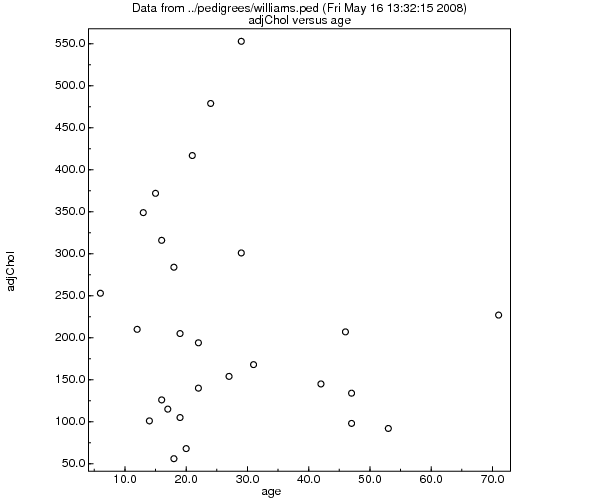# Sib-pair Command: plot

 Class Analysis and data manipulation command Name plot Arguments [].

Produce an Encapsulated Postscript scatterplot for two quantitative traits. A binary trait is scored as n=1 and y=2 for plotting purposes. If a third binary or quantitative trait is specified, then this controls the plot symbol used for each point. The trait values 1 to 10 are represented as:

circle=1, disc=2, square=3 upward_triangle=4 plus=5, diamond=6 cross=7 pentagon=8 downward_triangle=9 point=10

The graphic file name defaults to "sib-pair.eps".

Example:

```#
# Take advantage of macros to enhance plot command
# in a windowing environment.
# Primitive is still callable as "plo"
#
>> macro plot
plot>  plo %1 %2 %%.eps
plot>  \$ gv %%.eps
plot>  file delete %%.eps
plot> ;;;;
>> include williamsex.in